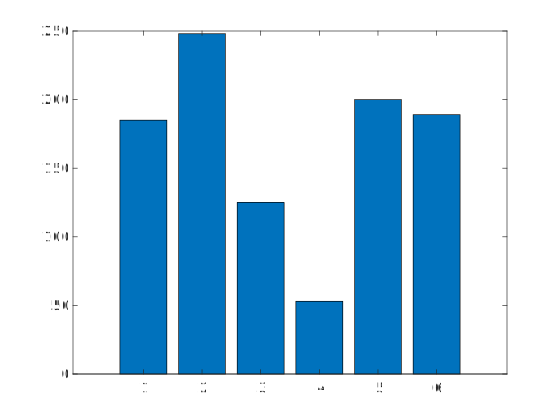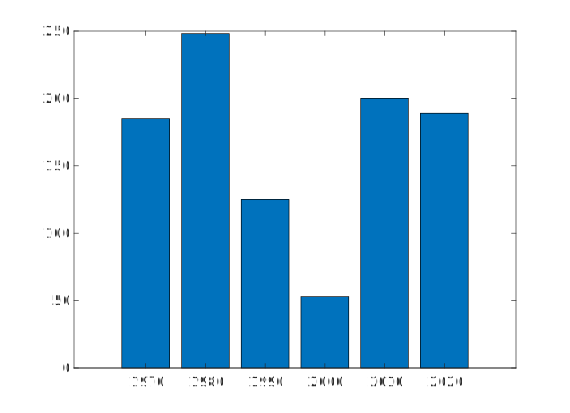Question #296

# In Matlab, how to draw a bar graph?

Merged questions

The MATLAB ﻿`bar()` function creates a bar graph in a figure. Let's consider the following vectors :

``````x = [1970 1980 1990 2000 2010 2020];
y = [185  248  125  53   200  189];``````

The following creates a bar graph with one bar for each element of ﻿`y`:

``﻿bar (y)``The following draws the bars at the location specified by ﻿`x`:

``bar(x,y);``4 events in history

Icons proudly provided by Friconix.